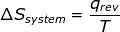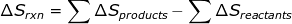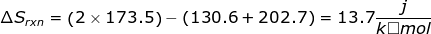# Entropy Formula

Entropy Formula

Entropy is a thermodynamic function used to measure the randomness or disorder of a system. For example, the entropy of a solid, where the particles are not free to move, is less than the entropy of a gas, where the particles will fill the container. Scientists have concluded that if a process is to be spontaneous, the entropy of that process must increase. This includes the entropy of the system and the entropy of the surroundings.

Entropy can be calculated using many different equations:

1. If the process is at a constant temperature then, where ΔS is the change in entropy, qrev is the reverse of the heat, and T is the Kelvin temperature.

2. If the reaction is known, then ΔSrxn can be calculated using a table of standard entropy values.3. Gibbs free energy (ΔG) and enthalpy (ΔH) can also be used to calculate ΔS.Entropy Formula Questions:1. Calculate the entropy change for the following reaction using the table of entropy values.

H2 (g) + F2 (g) → 2HF(g)2. Calculate the entropy change for the reaction using the table of entropy values.

2C4H10 (g) + 13O2 (g) → 8CO2 (g) + 10H2O(g)ΔSrxn = [(8 x 213.6) + (10 x 188.8)] - [(2 x 310.0) + (13 x 205.0)] = 311.8Related Links: Entropy and Gibbs Free Energy Quiz